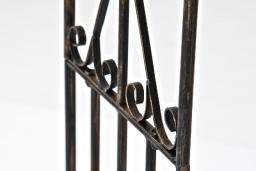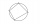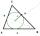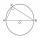# Inscribed triangle

To a circle is inscribed triangle so that the it's vertexes divide circle into 3 arcs. The length of the arcs are in the ratio 2:3:7. Determine the interior angles of a triangle.

α =  30 °
β =  45 °
γ =  105 °

### Step-by-step explanation:

$\beta =3a=45\text{°}=45\mathrm{°}$
$\gamma =7a=105\text{°}=105\mathrm{°}$Did you find an error or inaccuracy? Feel free to write us. Thank you!Tips to related online calculators

#### You need to know the following knowledge to solve this word math problem:

We encourage you to watch this tutorial video on this math problem:

## Related math problems and questions:

• Circumferential angleVertices of the triangle ΔABC lay on the circle and divided into arcs in the ratio 7:8:7. Determine the size of the angles of the triangle ΔABC.
• Interior anglesIn a quadrilateral ABCD, whose vertices lie on some circle, the angle at vertex A is 58 degrees and the angle at vertex B is 134 degrees. Calculate the sizes of the remaining interior angles.
• The chordThe side of the triangle inscribed in a circle is a chord passing through the circle center. What size are the internal angles of a triangle if one of them is 40°?
• Complete constructionConstruct triangle ABC if hypotenuse c = 7 cm and angle ABC = 30 degrees. / Use Thales' theorem - circle /. Measure and write down the length of legs.
• Inscribed circleCalculate the magnitude of the BAC angle in the triangle ABC if you know that it is 3 times less than the angle BOC, where O is the center of the circle inscribed in the triangle ABC.
• Angles of the triangleABC is a triangle. The size of the angles alpha, beta are in a ratio 4: 7. The angle gamma is greater than the angle alpha by a quarter of a straight angle. Determine angles of the triangle ABC.
• Interior anglesCalculate the interior angles of a triangle that are in the ratio 2: 3: 4.
• Inscribed circleXYZ is right triangle with right angle at the vertex X that has inscribed circle with a radius 5 cm. Determine area of the triangle XYZ if XZ = 14 cm.
• Construct rhombusConstruct rhombus ABCD if given diagonal length | AC | = 8cm, inscribed circle radius r = 1.5cm
• Hexagon in circleCalculate the radius of a circle whose length is 10 cm greater than the circumference of a regular hexagon inscribed in this circle.
• Angles in triangleThe triangle is ratio of the angles β:γ = 6:8. Angle α is 40° greater than β. What are the size of angles of the triangle?
• Eq triangle minus arcsIn an equilateral triangle with a 2cm side, the arcs of three circles are drawn from the centers at the vertices and radii 1cm. Calculate the content of the shaded part - a formation that makes up the difference between the triangle area and circular cuts
• Circle sectionEquilateral triangle with side 33 is inscribed circle section whose center is in one of the vertices of the triangle and the arc touches the opposite side. Calculate: a) the length of the arc b) the ratio betewwn the circumference to the circle sector and
• Angles in ratioThe size of the angles of the triangle are in ratio x: y = 7: 5 and the angle z is 42° lower than the angle y. Find size of the angles x, y, z.
• CircumscribingDetermine the radius of the circumscribed circle to the right triangle with legs 6 cm and 3 cm.
• RT - inscribed circleIn a rectangular triangle has sides lengths> a = 30cm, b = 12.5cm. The right angle is at the vertex C. Calculate the radius of the inscribed circle.
• Alfa beta gamaThe triangle's an interior angle beta is 10 degrees greater than the angle alpha and gamma angle is three times larger than the beta. Determine the size of the interior angles.